# Related Videos

## Solve

Go!
1
2
3
4
5
6
7
8
9
0
a
b
c
d
f
g
m
n
u
v
w
x
y
z
.
(◻)
+
-
×
◻/◻
/
÷
2

e
π
ln
log
log
lim
d/dx
Dx
|◻|
=
>
<
>=
<=
sin
cos
tan
cot
sec
csc

asin
acos
atan
acot
asec
acsc

sinh
cosh
tanh
coth
sech
csch

asinh
acosh
atanh
acoth
asech
acsch

### Videos### Master Solving a quadratic equation using special factoring techniques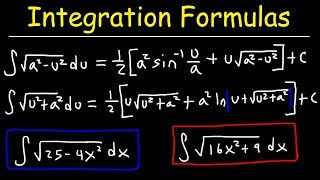### Integration Formulas For Trig Substitution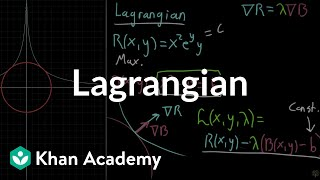### The Lagrangian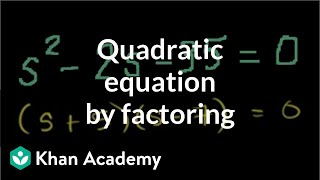### Solving a quadratic equation by factoring | Algebra II | Khan Academy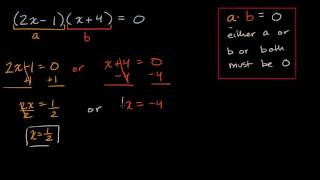### Solving equations with zero product property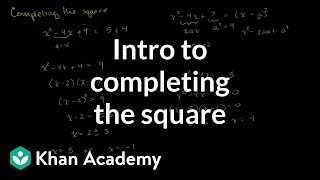### Solving quadratic equations by completing the square | Algebra II | Khan Academy

$\tan\left(x\right)+\cot\left(x\right)=\sec\left(x\right)\cdot\csc\left(x\right)$